## 1536 Reputation

11 years, 96 days

## Example...

Attached is an example of mine called Filters. The library is a module that contains all the functions you want in there.

You then need to save it where Maple can find it. As you can see, I pre-pended (in my maple.init file) my library directory to the others in libname; Maple will look in my own directory first before looking in its general directory (thus I can overlay my own definitions if I so chose). then you use LibraryTools:-Save to put the .mla file in there.

After that, with(Filters) (in my case) will find it.

I do agree with SGJanssens suggestion, but this example may get you going faster.

M.D.

Filters.mw

I think the closest to structs are records. They are related to modules but are more geared towards data structures, although you can store procedures as well. You can access members of a record using :- notation.

E.g.:

```Struct := Record(c=2*Pi*rho,a=Pi*rho^2);

Struct:-c;
2 π rho
Struct:-a;
π rho^2
```

Members can be Vectors, Arrays, you name it.

As for speed, Maple can be ok if you spend time programming it right. Naive implementation can lead to constant reallocation of memory, which gets costly. I am no expert on this although I have certainly run into unexpected super-linear growth of execution time with the number of cases. Declaring datatype=float (as opposed to "anything") in Vectors can help.

Look up the Maple Programming guide; a treasure trove of good practices.

I hope this helps,

Mac Dude

## Maybe this?...

You can use LinearAlgebra:-MatrixExponential(A) to evaluate exp(A).

You can also first Taylor-expand A and then substitute A:

```A=<<a11|a12>,<a21|a22>>;
exp(A);
taylor((3),A,3);
```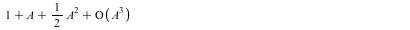```eval(taylor((3),A,3),A=<<a11|a12>,<a21|a22>>);
```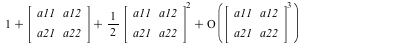```convert((5),polynom); # (5) is the above expression
```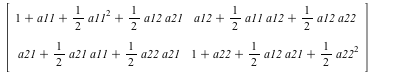The output of exp(A) is in the attached sheet, too big to print here

You'll have to decide whether the above does what you intend.

Mac Dude

exp_of_A.mw

## I corrected your expression by replacing...

I corrected your expression by replacing the square brackets [] with parentheses () and the &ndash; with - (minus sign). Your formula then reads like this:

f := x -> exp((-1)*0.13/x)(0.4700036292*`-`(0.3364722366, 1.2)) + exp(-1/x)(0.2135277634 + 0.528*x) + (-1)*0.528*x + 0.5420043550;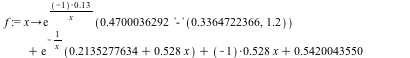Now you can try solve:
solve(f(x)=0,x);

and Maple replies

4.37165834535564

You can now check the result

f(4.37165834535564);

0.

I hope this helps,

M.D.

mp.mw

## cut & paste...

While not particularly elegant, I use cut & paste for such tasks. In fact, being a bit paranoid, I copy-paste the code out of the section first and then delete the original and the section.

After that, double-check your references if you referenced to any formulae in the moved sections of code. Usually Maple is good at keeping track of things, but in this case I think you have to update your references.

Mac Dude.

## frontend...

Use frontend to temporarily freeze the functions. The trick is that you need to specify the set of types to not freeze, the defaults (`+`,`*`) don't work as then the whole set is frozen. And -y(0) is of type `*` since it is -1*y(0), so you need to include that as well.

```frontend(fsolve,[{x(0)+y(0)=5,x(0)-y(0)=3},{x(0),y(0)}],[{`+`,`*`,`=`,'set'},{}]);
```

returns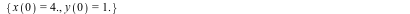You need to decide whether this is simpler & fits your purpose.

M.D.

fsolve-problem-frontend.mw

Edit: typos & concluding remark

I don't know what "CO2" in this context means (CO2 in my book is a gas, not a mass unit), but I am probably missing something here.

Anyway, the Units package has the AddUnits function that seems to me may be what you want:

```restart;with(Units);
convert(1.0,'units','m','Schritt');
1.25
A:=5*Unit('Schritt');
5 Schritt
combine(A,'units');
4 m
```

So in the "conversion" option you put the relation of your new unit to existing units. "combine" converts the custom unit to the default unit. "UseUnit()" sets the default unit to what you want. Note that AddUnit() can override existing units.

Note that the Units package has several systems it can work in (SI, cgs, etc.). You probably need to select the system before AddUnit.

I hope this helps,

M.D.

## One Way...

Here is one way to transfer info from Maple sheet A to Maple sheet B. The code snippets are from actual code I wrote and use.

In sheet A, you write out the items you need in sheet B. Note that these can be numbers or expressions. I put the left sides in quotes to avoid evaluation of the names.

```writestat("AlgParms.txt",'f__clk'=f__clk,'f__SR'=f__SR,'f__Boo__in'=f__Boo__in,'f__Boo__ex'=f__Boo__ex,\
'h__SR'=h__SR,'h__Boo'=h__Boo,'r'=r,'c__0'=c__0__act,'c__1'=c__1__act,'c__ex'=c__ex__act,'dpp__in'=dpp__in,'dpp__ex'=dpp__ex,\
'nbits'=n,'r'=r,'delta__W'=delta__W,'N__1'=N__1,'N__2'=N__2,'W__SR'=W__SR,'W__in'=W__in,'W__ex'=W__ex,\
'm'=eval(m,[c__0=c__0__act,c__1=c__1__act,c__ex=c__ex__act]),\
'mc'=eval(mc,[c__0=c__0__act,c__1=c__1__act,c__ex=c__ex__act]),\
'F__c'=eval(rhs((8.6)),[c__0=c__0__act,c__1=c__1__act,c__ex=c__ex__act]),\
'W'=eval(rhs((9.12)),[c__0=c__0__act,c__1=c__1__act,c__ex=c__ex__act])):
close("AlgParms.txt"):

```

Note that some of these are eval statements.

In sheet B, you read-in the file:

```FileTools:-Text:-ReadFile("AlgParms.txt");
assign(parse(%));close("AlgParms.txt");
```

at which point you have all the variables assigned as they are given in the file. In the example many were expressions.

This works for small-ish data quantities. I remember I ran into issues when trying to use the same mechanism for large quantities (rtables, tables, modules and such) where the info just would not make it across. If you look up a previous question of mine here on MaplePrimes ("How can I save a Record...") then you can read through a whole thread where acer is explaining how to do that using .mla files. I did struggle with that (as is evident from the thread) but eventually I did get it to work.

I hope this helps,

M.D.

## Kernel crashed...

Maple has a front-end (user interface, the program you interact with) and a kernel that executes the statements you enter. These are separate processes. Usually the connection-lost message indicates that the kernel crashed. The question then is "Why would it do that?"

The output you get indicates rather beefy RAM usage: 32 GB allocated and about a TB used??  That sounds like an endless loop to me that you let run for a long time. So you need to go back to your code & put in a few strategic print() statements to see if it works as expected. Note that you can limit the time it runs if you don't want to wait until it dies; alternately, since you use the command line, ^C is your friend (Don't run it with nohup and do not detach your terminal).

If it gets hung in the Groebner Basis calculation, then you need to check whether the input to that is as expected. Beyond that I cannot help, not being familiar with the Groebner package.

M.D.

Loading a package using with() masks the global definition of any symbol you export in the package. I once wrote a package that redefined the same dot operator you use, `.`. As a result the global definition of `.` no longer was effective & I had to revert to a messy construction using overload() to apply my redefinition only to the types that needed it. So the behaviour you see seems to me as expected. Do note that VectorCalculus:-`.` could be written such that it preserves the functionality of :-`.` by clever way of using type definitions. In that sense, VectorCalculus:-`.` indeed can check its arguments & fall back on using the normal :-`.` where applicable. I hasten to add that I don't know what VectorCalculus:-`.` is actually doing in this regard.

I have been wondering about the effect of name clashes myself, when several packages redefine the same symbol. So just as you are, I am hesitant loading multiple packages using with(). It should not be difficult to test Maple's behaviour in such cases. I'd speculate that using several with() statements, each in its own execution group, the last one wins.

M.D.

## Yes, this does happen, and it can be mig...

Yes, this does happen, and it can be mighty annoying as all variable values are gone & one cannot find out what went wrong. There is no good way around this I know; but a few hints:

(a): Save a sheet before you attempt to run it. In this way you preserve your starting point.

(b): If this happens in a loop, lower the end point so that it only does a very limited number of iterations. Print the index at each iteration so you know how far it gets.

(c): Check the memory value at the bottom of the sheet. If it goes up and up you are likely running into "expression swell", i.e. some intermediate expressions are growing, most likely exponentially. Terminate the process in the early stages so you can get an idea which ones are growing. Occasionally (but not very often) I have had luck with running simplify at each step. If you use modules and Records you may run into a memory leak, i.e. Maple keeps allocating space for new objects which will make it run out of memory while at the same time slow it to a crawl. This situation also reveals itself in an increase in time per iteration if a loop is involved (which is usually the case).

(d): Post an example here that shows this behaviour. Mention the Maple version you run.

There are ways to limit space and time but I don't know whether they preserve variables. Check help on kernelopts() for these.

M.D.

## plots:-setoptions...

plots:-setoptions(size=[1200,500]) in your .mapleinit file will make this the default for 2d plots.

I do note that I have had on occasion issues with setoptions, so it is conceivable that for thing like plots:-display() the option might be ignored.

Mac Dude

Edit: You can set most option with this command. See the Help on setoptions.

## f is overwritten near the end of the loo...

f is overwritten near the end of the loop; the new f with no more c[i] is then used in eq1 on the 2nd pass  through the loop. Then the fsolve returns NULL (no variables to solve for) and the eval fails. If this is intended then you have a problem. If f is always to have the form as in the assignment after eq1, then assign the eval to g near the bottom and plot g. That produces this plot: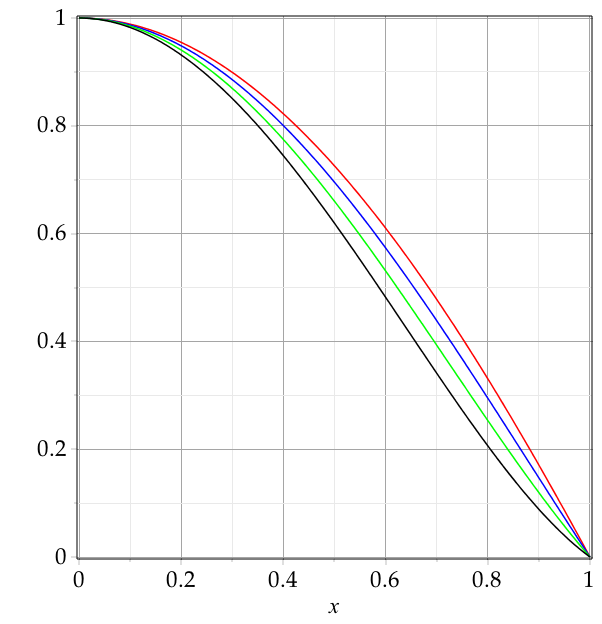Mac Dude

DAM(1)u.mw

## One way is to eval your series: eval(se...

One way is to eval your series:

eval(series(...),g='x->x^2+1'); # note the uneval quotes

which returns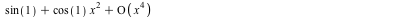This is of type series, to make it a normal expression use

convert(serr,'polynom');

to get rid of the O(x^4) part.

M.D.

## Maple Programming guide; Emacs maplev mo...

Well, there is the Maple Programming Guide; an excellent document describing Maple programming in detail. I learned a lot from it and still use it for reference when needed.

As for editing, I suggest looking into the maplev Emacs module by Joe Riel. This gives you syntax coloring, indentation and such. You edit a .mpl file with Maple code; that you then read into the worksheet to execute it. Or, if you don't need the graphical aspects of Maple's GUI, you can run it directly from emacs using the command-line version of Maple. What I personally do is to put code of any length or significance into a package edited using emacs/maplev an then read the package & save it as .mla file using LibraryTools.

A debugger is available in the standard GUI. stopat(routineame) will bring it up.

M.D.

﻿